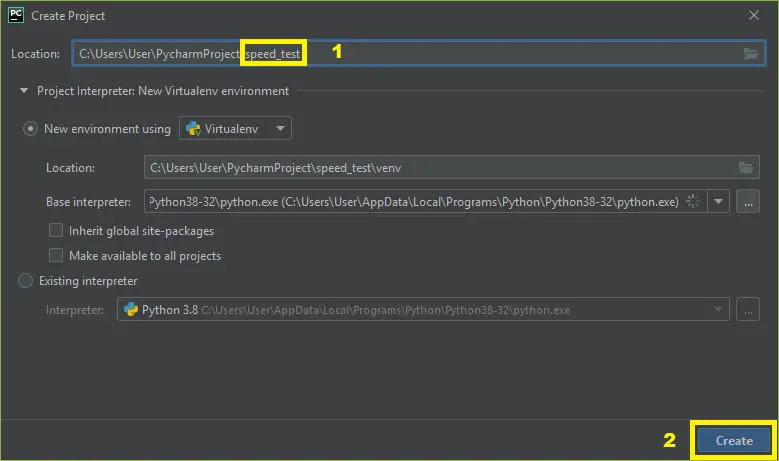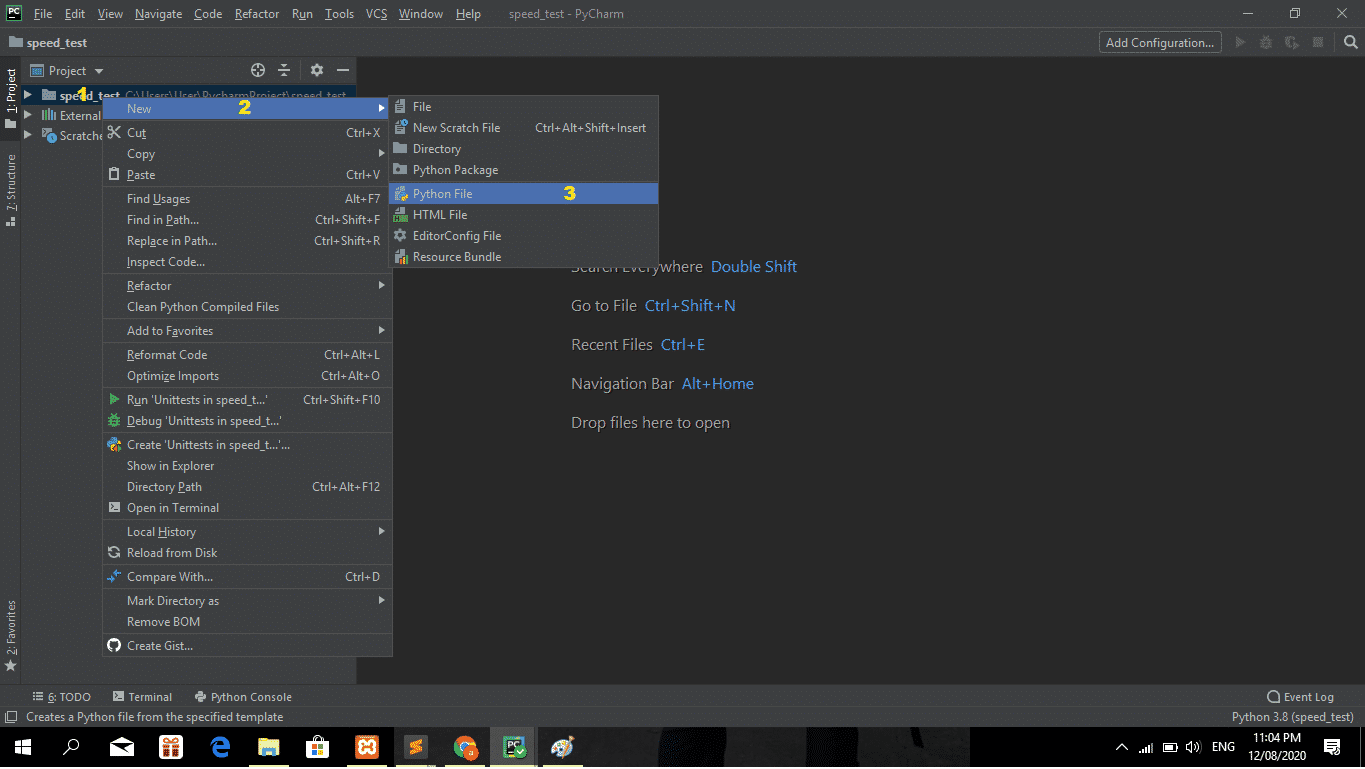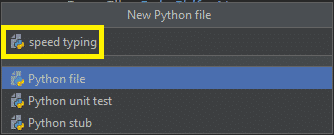# Python Speed Typing Test With Free Source Code

The Python Speed Typing Test is written in Python programming language, Speed Typing Test Python project idea, we are going to build an exciting project through which you can check and even improve your typing speed.

To create a graphical user interface(GUI), we are going to use the pygame library which is used for working with graphics.

A Python Typing Speed Test is good for students or beginners to improve their typing test skills.

This Python Typing Game is good for the beginners in Python programming world because the syntax is easy to learn and understand.

## Python Project on Typing Speed Test: Project Information

Anyway, if you want to level up your knowledge in programming especially games in Python, try this new article I’ve made for you Code For Game in Python: Python Game Projects With Source Code.

To start creating a Speed Typing Test Python Project, make sure that you have PyCharm IDE installed on your computer.

By the way, if you are new to Python programming and don’t know what Python IDE is and how to use it, I have here a list of the Best Python IDE for Windows, Linux, and Mac OS that will suit you.

## How to Create a Python Project on Speed Typing Test

Time needed: 5 minutes

These are the steps to create a Python Speed Typing Test with Source Code

• Step 1: Create a project name.

First, open Pycharm IDE and then create a “project name.” After creating a project name click the “create” button.• Step 2: Create a python file.

Second, after creating a project name, “right-click” your project name and then click “new.” After that click the “python file“.• Step 3: Name your python file.

Third, after creating a Python file, Name your Python file after that click “enter“.• Step 4: The actual code.

You are free to copy the code given below and download the full source code below.

## Code Explanations

### 1. The Code Given Below Is For Importing Modules

Code:

``````import pygame
from pygame.locals import *
import sys
import time
import random``````

Explanation:

The code is given which is importing all modules.

### 2. The Code Given Below Is For The Class Game

Code:

``````class Game:

def __init__(self):
self.w=750
self.h=500
self.reset=True
self.active = False
self.input_text=''
self.word = ''
self.time_start = 0
self.total_time = 0
self.accuracy = '0%'
self.results = 'Time:0 Accuracy:0 % Wpm:0 '
self.wpm = 0
self.end = False
self.TEXT_C = (240,240,240)
self.RESULT_C = (255,70,70)

pygame.init()
self.open_img = pygame.transform.scale(self.open_img, (self.w,self.h))

self.bg = pygame.transform.scale(self.bg, (500,750))

self.screen = pygame.display.set_mode((self.w,self.h))
pygame.display.set_caption('Type Speed test')
``````

Explanation:

In this class, we initialized the width and height of the window and variables that are needed for calculation, and then we initialized the pygame and loaded the images. The screen variable is the most important on which we will draw everything.

### 3. The Code Given Below Is For The Module draw_text

Code:

``````    def draw_text(self, screen, msg, y ,fsize, color):
font = pygame.font.Font(None, fsize)
text = font.render(msg, 1,color)
text_rect = text.get_rect(center=(self.w/2, y))
screen.blit(text, text_rect)
pygame.display.update() ``````

Explanation:

This module declares the font, text, size, and color of the screen.

### 4. The Code Given Below Is For The Module get_sentence

Code:

``````    def get_sentence(self):
sentences = f.split('\n')
sentence = random.choice(sentences)
return sentence``````

Explanation:

In this module which is getting the data sentence in a text file, to be performed in a text field.

### 5. The Code Given Below Is For The Module show_results

Code:

``````    def show_results(self, screen):
if(not self.end):
#Calculate time
self.total_time = time.time() - self.time_start

#Calculate accuracy
count = 0
for i,c in enumerate(self.word):
try:
if self.input_text[i] == c:
count += 1
except:
pass
self.accuracy = count/len(self.word)*100

#Calculate words per minute
self.wpm = len(self.input_text)*60/(5*self.total_time)
self.end = True
print(self.total_time)

self.results = 'Time:'+str(round(self.total_time)) +" secs   Accuracy:"+ str(round(self.accuracy)) + "%" + '   Wpm: ' + str(round(self.wpm))

# draw icon image
self.time_img = pygame.transform.scale(self.time_img, (150,150))
#screen.blit(self.time_img, (80,320))
screen.blit(self.time_img, (self.w/2-75,self.h-140))
self.draw_text(screen,"Reset", self.h - 70, 26, (100,100,100))

print(self.results)
pygame.display.update()``````

Explanation:

This module shows the results of your typing test, which depends on your typing speed.

### 6. The Code Given Below Is For The Module run

Code:

``````    def run(self):
self.reset_game()

self.running=True
while(self.running):
clock = pygame.time.Clock()
self.screen.fill((0,0,0), (50,250,650,50))
# update the text of user input
self.draw_text(self.screen, self.input_text, 274, 26,(250,250,250))
pygame.display.update()
for event in pygame.event.get():
if event.type == QUIT:
self.running = False
sys.exit()
elif event.type == pygame.MOUSEBUTTONUP:
x,y = pygame.mouse.get_pos()
# position of input box
if(x>=50 and x<=650 and y>=250 and y<=300):
self.active = True
self.input_text = ''
self.time_start = time.time()
# position of reset box
if(x>=310 and x<=510 and y>=390 and self.end):
self.reset_game()
x,y = pygame.mouse.get_pos()

elif event.type == pygame.KEYDOWN:
if self.active and not self.end:
if event.key == pygame.K_RETURN:
print(self.input_text)
self.show_results(self.screen)
print(self.results)
self.draw_text(self.screen, self.results,350, 28, self.RESULT_C)
self.end = True

elif event.key == pygame.K_BACKSPACE:
self.input_text = self.input_text[:-1]
else:
try:
self.input_text += event.unicode
except:
pass

pygame.display.update()

clock.tick(60)``````

Explanation:

This module which is the main module of our class will handle all the events.

### 7. The Code Given Below Is For The Module reset_game

Code:

``````    def reset_game(self):
self.screen.blit(self.open_img, (0,0))

pygame.display.update()
time.sleep(1)

self.reset=False
self.end = False

self.input_text=''
self.word = ''
self.time_start = 0
self.total_time = 0
self.wpm = 0

# Get random sentence
self.word = self.get_sentence()
if (not self.word): self.reset_game()
self.screen.fill((0,0,0))
self.screen.blit(self.bg,(0,0))
msg = "Typing Speed Test"
# draw the rectangle for input box
pygame.draw.rect(self.screen,(255,192,25), (50,250,650,50), 2)

# draw the sentence string
self.draw_text(self.screen, self.word,200, 28,self.TEXT_C)

pygame.display.update()``````

Explanation:

This module which is resets all variables so that we can start testing our typing speed again.

## Complete Source Code

``````import pygame
from pygame.locals import *
import sys
import time
import random

# 750 x 500

class Game:

def __init__(self):
self.w=750
self.h=500
self.reset=True
self.active = False
self.input_text=''
self.word = ''
self.time_start = 0
self.total_time = 0
self.accuracy = '0%'
self.results = 'Time:0 Accuracy:0 % Wpm:0 '
self.wpm = 0
self.end = False
self.TEXT_C = (240,240,240)
self.RESULT_C = (255,70,70)

pygame.init()
self.open_img = pygame.transform.scale(self.open_img, (self.w,self.h))

self.bg = pygame.transform.scale(self.bg, (500,750))

self.screen = pygame.display.set_mode((self.w,self.h))
pygame.display.set_caption('Type Speed test')

def draw_text(self, screen, msg, y ,fsize, color):
font = pygame.font.Font(None, fsize)
text = font.render(msg, 1,color)
text_rect = text.get_rect(center=(self.w/2, y))
screen.blit(text, text_rect)
pygame.display.update()

def get_sentence(self):
sentences = f.split('\n')
sentence = random.choice(sentences)
return sentence

def show_results(self, screen):
if(not self.end):
#Calculate time
self.total_time = time.time() - self.time_start

#Calculate accuracy
count = 0
for i,c in enumerate(self.word):
try:
if self.input_text[i] == c:
count += 1
except:
pass
self.accuracy = count/len(self.word)*100

#Calculate words per minute
self.wpm = len(self.input_text)*60/(5*self.total_time)
self.end = True
print(self.total_time)

self.results = 'Time:'+str(round(self.total_time)) +" secs   Accuracy:"+ str(round(self.accuracy)) + "%" + '   Wpm: ' + str(round(self.wpm))

# draw icon image
self.time_img = pygame.transform.scale(self.time_img, (150,150))
#screen.blit(self.time_img, (80,320))
screen.blit(self.time_img, (self.w/2-75,self.h-140))
self.draw_text(screen,"Reset", self.h - 70, 26, (100,100,100))

print(self.results)
pygame.display.update()

def run(self):
self.reset_game()

self.running=True
while(self.running):
clock = pygame.time.Clock()
self.screen.fill((0,0,0), (50,250,650,50))
# update the text of user input
self.draw_text(self.screen, self.input_text, 274, 26,(250,250,250))
pygame.display.update()
for event in pygame.event.get():
if event.type == QUIT:
self.running = False
sys.exit()
elif event.type == pygame.MOUSEBUTTONUP:
x,y = pygame.mouse.get_pos()
# position of input box
if(x>=50 and x<=650 and y>=250 and y<=300):
self.active = True
self.input_text = ''
self.time_start = time.time()
# position of reset box
if(x>=310 and x<=510 and y>=390 and self.end):
self.reset_game()
x,y = pygame.mouse.get_pos()

elif event.type == pygame.KEYDOWN:
if self.active and not self.end:
if event.key == pygame.K_RETURN:
print(self.input_text)
self.show_results(self.screen)
print(self.results)
self.draw_text(self.screen, self.results,350, 28, self.RESULT_C)
self.end = True

elif event.key == pygame.K_BACKSPACE:
self.input_text = self.input_text[:-1]
else:
try:
self.input_text += event.unicode
except:
pass

pygame.display.update()

clock.tick(60)

def reset_game(self):
self.screen.blit(self.open_img, (0,0))

pygame.display.update()
time.sleep(1)

self.reset=False
self.end = False

self.input_text=''
self.word = ''
self.time_start = 0
self.total_time = 0
self.wpm = 0

# Get random sentence
self.word = self.get_sentence()
if (not self.word): self.reset_game()
self.screen.fill((0,0,0))
self.screen.blit(self.bg,(0,0))
msg = "Typing Speed Test"
# draw the rectangle for input box
pygame.draw.rect(self.screen,(255,192,25), (50,250,650,50), 2)

# draw the sentence string
self.draw_text(self.screen, self.word,200, 28,self.TEXT_C)

pygame.display.update()

Game().run()

``````

## Summary

The Speed Typing Test Python Project is written in Python programming language, Python is very easy to research the syntax emphasizes readability and it is able to reduce time ingesting in developing.

Also, this tutorial is the simplest way for beginners or students to enhance their logical skills in programming. Aside from that, this game project is a way for students or beginners to design and develop games.

In this article, you worked on the Python project to build your own game of typing speed testing with the help of the pygame library.

I hope you got to learn new things and enjoyed building this interesting Python project. Do share the article on social media with your friends and colleagues.

## Inquiries

1.